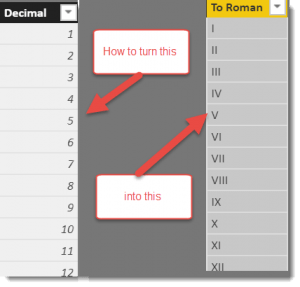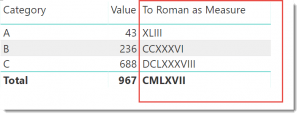# Quick DAX : Convert to Roman NumerialsOne of the biggest holes in DAX today is the lack of a native function that allows you to convert a decimal number to its roman number equivalent.  Excel has a ROMAN() function so I thought it was about time to share one approach to converting decimal numbers to Roman.  I can feel a collective sigh out in the DAX community at this post.   Seriously though, while this post is next to useless in practical terms, it does showcase how you might solve a tricky conversion problem in DAX using pivot/unpivot and nested SWITCH functions to keep your code light,

There are a few tricks in the Roman numbering system, particularly around the subtractive pattern (IV instead of IIII) to avoid excessive use of repeated characters.

To refresh your memory, the numbering system uses the following rules:

 Symbol Value I V X L C D M 1 5 10 50 100 500 1,000

For this PBIX file, I start by creating a calculated table with a single column carrying numbers between 1 and 3999.  I don’t address negative or numbers greater than 4000. I then add the following calculated column to the table that converts every number to its roman number equivalent.

```To Roman =
VAR StartValue = 'Numbers'[Decimal]
VAR TextLength = LEN(StartValue)
VAR GetComponents =
GENERATE(
ROW("MyValue",StartValue) ,
GENERATESERIES(1,TextLength)
),
"Pos",INT(MID([MyValue],TextLength-[Value]+1,1)))

"X" ,
SWITCH(
TRUE(),
[Pos]=9 , MID("IXCM",[Value],2),
[Pos]=4 , MID("IVXLCD",([Value]*2)-1,2),
[Pos]<4 , REPT(SWITCH([Value],1,"I",2,"X",3,"C",4,"M",""),[Pos]) ,
[Pos]<9 , SWITCH([Value],1,"V",2,"L",3,"D","") & REPT(SWITCH([Value],1,"I",2,"X",3,"C",4,"M",""),[Pos]-5) ,
""
))
RETURN
CONCATENATEX(
ToRoman,[X],,[Value],DESC)```

The code begins by assigning the number to be converted to the StartValue variable.  A table expression is built to split the number into significant numbers which are then processed using the ToRoman variable.

The final RETURN statement uses one of my favourite functions, CONCATENATEX to sew the numbers back into a single piece of text.

While this code is based on a calculated column, it could easily be converted to a calculated measure with the only tweak being to the line that assigns a value assigned to the StartValue variable.  I have also included a calculated measure version of this in the PBIX file.Next Quick DAX blog : how to convert roman numbers back so you can do maths like “LIV” + “LXXX” = “CXXXIV”

Phil is Microsoft Data Platform MVP and an experienced database and business intelligence (BI) professional with a deep knowledge of the Microsoft B.I. stack along with extensive knowledge of data warehouse (DW) methodologies and enterprise data modelling. He has 25+ years experience in this field and an active member of Power BI community.